You are using an outdated browser. Please upgrade your browser to improve your experience.## Simple Harmonic Motion Calculator are physic/math calculator to find amplitude of oscillations, angular frequency, frequency, time point to measure the displacement, displacement, velocity or acceleration fast and easy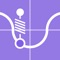# Simple Harmonic Motion Calc

by Nitrio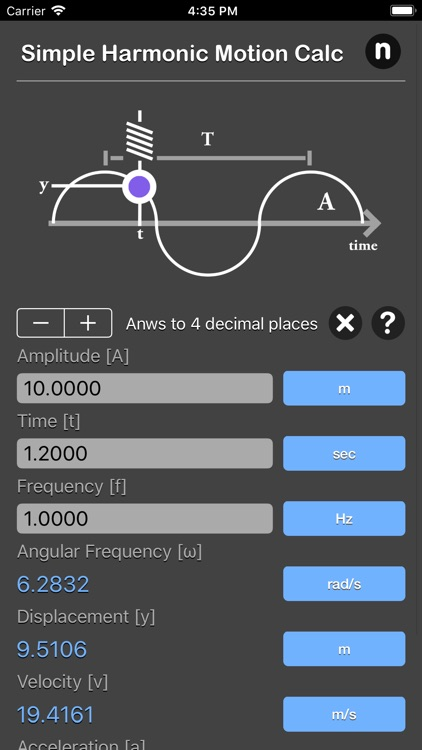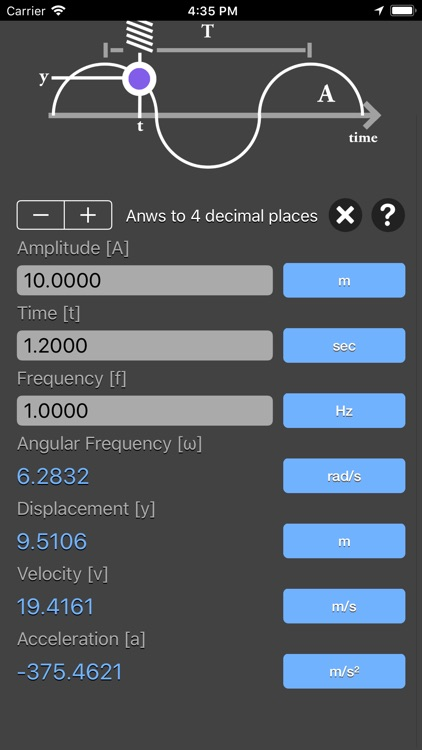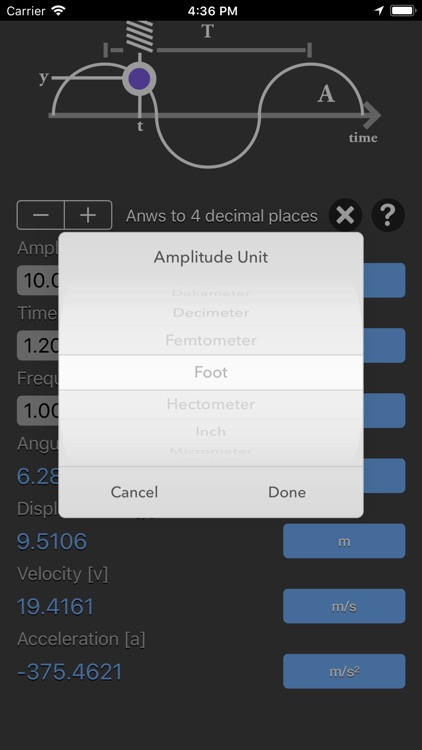### What is it about?

Simple Harmonic Motion Calculator are physic/math calculator to find amplitude of oscillations, angular frequency, frequency, time point to measure the displacement, displacement, velocity or acceleration fast and easy.### App Details

Version
1.0
Rating
NA
Size
15Mb
Genre
Productivity Utilities
Last updated
July 27, 2018
Release date
July 7, 2018

### App Screenshots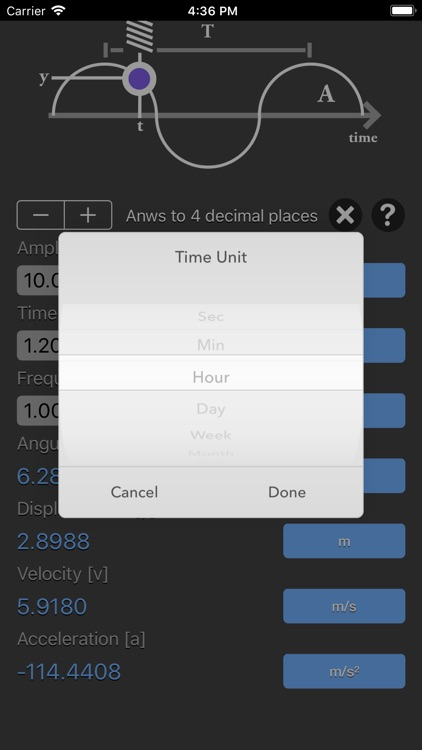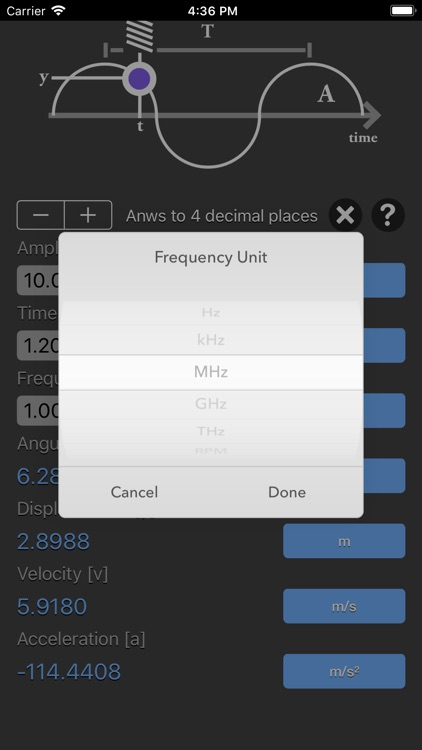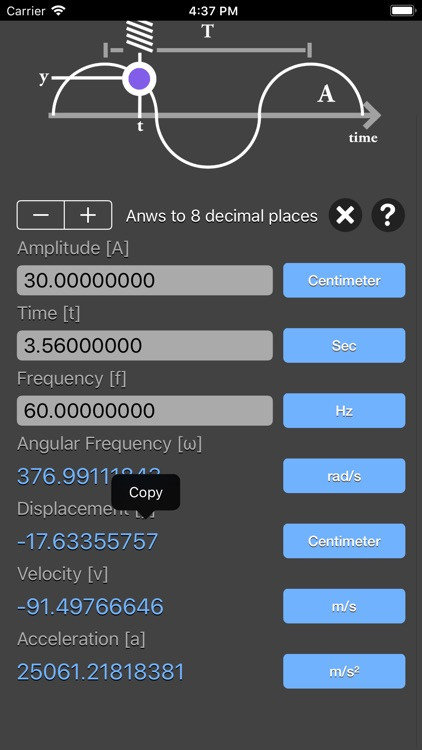### App Store Description

Simple Harmonic Motion Calculator are physic/math calculator to find amplitude of oscillations, angular frequency, frequency, time point to measure the displacement, displacement, velocity or acceleration fast and easy.

Features:
- Instant calculation
- Result are copy able to other app
- Formula are include as reference
- Support up to 16 decimal place
- Support various unit for each input

In mechanics and physics, simple harmonic motion is a special type of periodic motion or oscillation motion where the restoring force is directly proportional to the displacement and acts in the direction opposite to that of displacement.

Simple harmonic motion can serve as a mathematical model for a variety of motions, such as the oscillation of a spring. In addition, other phenomena can be approximated by simple harmonic motion, including the motion of a simple pendulum as well as molecular vibration. Simple harmonic motion is typified by the motion of a mass on a spring when it is subject to the linear elastic restoring force given by Hooke's Law. The motion is sinusoidal in time and demonstrates a single resonant frequency. For simple harmonic motion to be an accurate model for a pendulum, the net force on the object at the end of the pendulum must be proportional to the displacement. This is a good approximation when the angle of the swing is small.

simple harmonic motion equations:
y = A * sin(ωt)
v = A * ω * cos(ωt)
a = - A * ω² * sin(ωt)
ω = 2πf

where:
A is the amplitude of oscillations
ω is the angular frequency of oscillations in rad/s
f is the frequency
t is the time point when you measure the particle's displacement
y is the displacement
v is the velocity,
a is the acceleration

*This is a universal app that work for both iPhone and iPad.

Thanks for your support and do visit nitrio.com for more apps for your iOS devices.

Disclaimer:
AppAdvice does not own this application and only provides images and links contained in the iTunes Search API, to help our users find the best apps to download. If you are the developer of this app and would like your information removed, please send a request to [email protected] and your information will be removed.